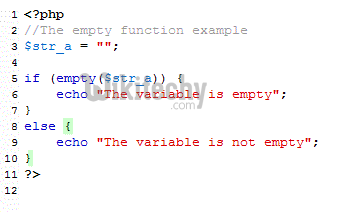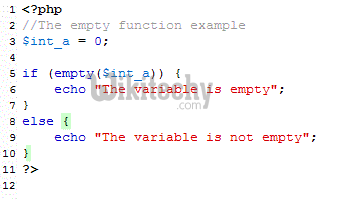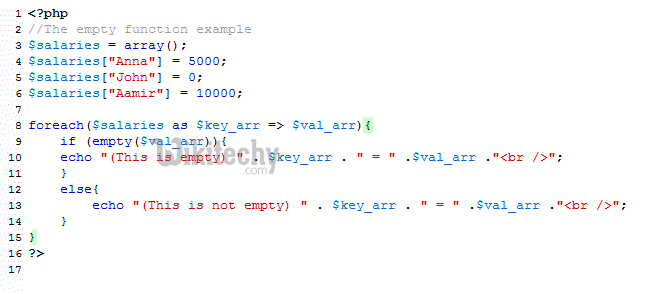# php tutorial - PHP Empty Function - php programming - learn php - php code - php script

## What is PHP empty functions - Purpose of empty function

• The PHP empty function is used to determine if a variable is empty or not.
• It will return True if the given variable is empty and false if it exists or a non-zero or more than zero characters etc.
• I will explain further in the later part of this tutorial, first syntax and live examples of using the empty function.
• ## Syntax to use empty function

• This is how you can use the empty PHP function:
• Where, the variables can be of different types like an integer, string or even an array etc.
• ## An example of using the empty function

• In the following example, a string variable is declared and given a zero character value, i.e. “”. After that, the if condition is used with the empty function.
• See the code and output by clicking the link below:
•• You can see, the variable is shown as empty. That means if a string variable is declared and even assigned a “” value it is still taken as empty. However, if it contains some value like “abc”, it will not be considered as empty.
• ## An example with integer type variable

• In this example, an integer type variable is declared and assigned a 0 value. See the output and description below:
•• So, it still returned as true or the integer variable is taken as empty. If the variable contains 1 or more value it will not be taken as empty, in the case of a string or float variable.
• ## An empty example with array element

• In this example an associative array of three elements is used. The first and third elements of the array contain value other than zero. While the second element is assigned the 0 value. See the output yourself by clicking the link or image below:
•• You see, the foreach loop is used to loop through the array elements. Inside the foreach, the if statement is also used and a message is displayed if the current array element is empty or not.
• The second element is shown as empty while other two as non-empty.
• ## Why we use the empty function?

• Sometimes, you need to check if a variable contains a value or not before proceeding to some action.
• For example, you are taking the input values from the user in a web form. A few fields like Name, email, address are mandatory in the form. While you can check it at the client side by using the JavaScript, however, sometimes it may be disabled.
• In that case, you may check the variable values by using the empty function to ensure that a user has entered some values or otherwise redirect the user back to the form page rather than proceeding to save the information in the database.
• ## How to use PHP empty function

• Using the empty function is quite simple. You simply need to provide a variable name to check if it contains a value or is it is empty.
• This is what the empty function takes as ‘empty’:
• If your string contains no characters or it is like this: Str = “”;
• Then this will be considered as empty.
• If a variable is not defined or does not exist, it will be considered as empty.
• If you have declared a variable and not given any value, this will still be considered as empty.
• If a Boolean variable is False, this is also considered as empty.
• The integer or float variable with 0 value are also considered as empty.
• A variable with NULL value is also taken as empty.
• An empty array is also taken as “empty”.